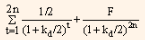## Valuing semi-annual cash flows, Financial Management

Assignment Help:

In all previous illustrations, we assumed that coupon payments are paid on annual basis. However, most of the bonds carry interest payment semi-annually. Semi-annual coupon payments can be calculated by dividing the annual coupon payment and discount rate by 2. The time period n in the present value formula is treated in terms of 6-month period rather than years.

For example consider a 7%, 5-year bond with a discount rate of 6% and a maturity value of Rs.100. The cash flow, in the form of interest, for the first four and half years will be Rs.3.50 (Rs. 7/2) and last cash flow will be Rs.103.50, i.e., interest of Rs.3.50 and a principal of Rs.100.

The annual discount rate is 6% so semi-annual discount rate will be 3% (6% / 2).

When coupon rate is 7%, the semi-annual coupon rate is 3.5%. Then PV of cash flow will be:

Table 1: Calculation of PV of 7% Bond when Cash Flows are Semi-annual

 Year Cash Flow (in Rs.) PV (in Rs.) I half 2007 3.5 3.40 II half 2007 3.5 3.30 I half 2008 3.5 3.20 II half 2008 3.5 3.11 I half 2009 3.5 3.02 II half 2009 3.5 2.93 I half 2010 3.5 2.85 II half 2010 3.5 2.76 I half 2011 3.5 2.68 II half 2011 103.5 77.01 Present Value = 104.27

If we compare the PV of table 1 then we find that PV of table 1 is greater by Rs.0.06. This is because one-half the annual coupon payment is received six months sooner than when payments are annual.

We can divide the value of non-amortizing bond into two parts, first is PV of coupon payment and second is PV of maturity value. For fixed coupon rate, coupon payments represent an annuity. A short-cut formula for computing the value of bond when coupon rate is fixed and single discount rate is set for discounting the coupon payments, is to compute the PV of the annuity and then add the PV of maturity value.  We can represent it in formula as follows:

V0      ==       I ¤ 2 (PVIFAkd ¤ 2,2n) + F(PVIFkd/2, 2n)                                           ... Eq. (3)

Where,

V        =    value of the bond

I/2      =    semi-annual interest payment

F         =    par value of the bond payable at maturity

kd/2    =     required rate of return for the half-year period

2n        =     maturity period expressed in half-yearly periods.

#### State the importance of gearing in accounting, state the importance of gear...

state the importance of gearing in accounting Gearing is one of the most extensively used terms in accounting. Gearing is the relationship between debt and equitywhich means th

#### Beta value, Beta Value Risk is an important consideration while investi...

Beta Value Risk is an important consideration while investing in any security. It is the possibility that realised returns will be less than the returns expected. The degree, t

#### Determine the optimal production quantity, Water Wheelies manufactures high...

Water Wheelies manufactures high-pressure sprinkler heads. These are produced periodically at a rate of 20,000 per month. Demand is steady at 15,000 per month. Each production run

#### Differences in working capital for different industries, Differences in wor...

Differences in working capital for different industries   Manufacturing Retail Service Inventories H

#### Debt securities , lso from the auditor's report, they have reported that th...

lso from the auditor's report, they have reported that the company has used funds raised on short-term basis for long-term investment. The company has purchased certain fixed asses

#### Futures and forward, what factors influence the decision to use futures or ...

what factors influence the decision to use futures or forwards contracts

#### Determine about the sales agents, Determine about the Sales agents Norm...

Determine about the Sales agents Normally used for more effective sales and marketing activities for a product for example AVON (cosmetics) door to door agents in the UK. -

calculate npv

#### Validity of the accounting implications, Question: In each case below a...

Question: In each case below and having regard to your knowledge of Accounting Concepts, comment on and assess the validity of the accounting implications/practices to be adop

#### Hedging using commodity futures, Hedging Using Commodity Futures Produc...

Hedging Using Commodity Futures Producers of agricultural commodities are faced with price risk and production risk over a period of time and within a marketing year. In case o